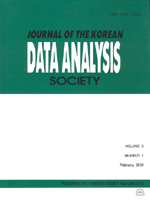상세검색
최근 검색어 전체 삭제
다국어입력
즐겨찾기0KCI등재 학술저널

# Modified Confidence Intervals by Broemeling s Method in Two-Factor Nested Variance Components Model

• 등재여부 : KCI등재
• 2008.06
• 1301 - 1312 (12 pages)

The confidence intervals for (수식) are given in various forms by many authors in two-factor nested variance components model (수식). In this model, the confidence intervals by using Broemeling s method for (수식) are given by an asymptotic confidence intervals. By the simulations results of this confidence coefficients are not fit exactly the given confidence coefficient 1-alpha. Thus, we would like to give one of the improved confidence intervals for (수식)2 by using Broemeling s method in two-factor nested variance components model.

1. Introduction

2. Two-Factor Nested Variance Components Model

3. Confidence Intervals

4. Simulation Results

References